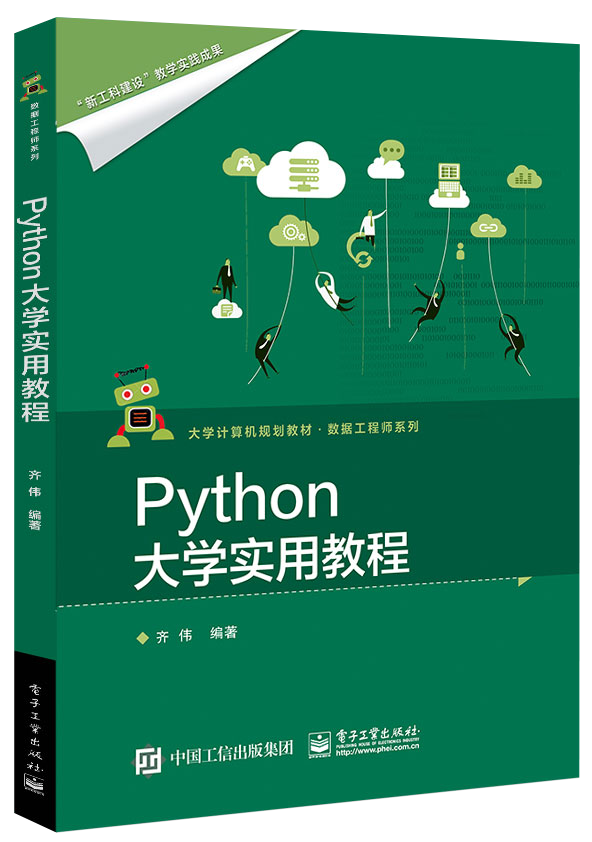# Python初学者应该了解的星号（*）

• Python• 作为乘法和乘方的运算符
• 表示序列中元素的重复
• 用于收集参数（可以称之为“打包”）
• 用于容器类对象的解包

## 乘法或乘方的运算符

>>> 2 * 3
6
>>> 2 ** 3
8
>>> 1.414 * 1.414
1.9993959999999997
>>> 1.414 ** 1.414
1.6320575353248798


## 重复类列表的容器元素

Python也支持类列表的容器类对象（即序列）与整数相乘，即为按照整数实现重复其中的元素数量。

# Initialize the zero-valued list with 100 length
zeros_list =  * 100

# Declare the zero-valued tuple with 100 length
zeros_tuple = (0,) * 100

# Extending the "vector_list" by 3 times
vector_list = [[1, 2, 3]]
for i, vector in enumerate(vector_list * 3):
print("{0} scalar product of vector: {1}".format((i + 1), [(i + 1) * e for e in vector]))
# 1 scalar product of vector: [1, 2, 3]
# 2 scalar product of vector: [2, 4, 6]
# 3 scalar product of vector: [3, 6, 9]


## 参数收集

# A function that shows the results of running competitions consisting of 2 to 4 runners.
def save_ranking(first, second, third=None, fourth=None):
rank = {}
rank, rank = first, second
rank = third if third is not None else 'Nobody'
rank = fourth if fourth is not None else 'Nobody'
print(rank)

# Pass the 2 positional arguments
save_ranking('ming', 'alice')
# Pass the 2 positional arguments and 1 keyword argument
save_ranking('alice', 'ming', third='mike')
# Pass the 2 positional arguments and 2 keyword arguments (But, one of them was passed as like positional argument)
save_ranking('alice', 'ming', 'mike', fourth='jim')


def save_ranking(first, second=None, third, fourth=None):
...


### 收集位置参数

def save_ranking(*args):
print(args)
save_ranking('ming', 'alice', 'tom', 'wilson', 'roy')
# ('ming', 'alice', 'tom', 'wilson', 'roy')


### 收集位置参数和关键词参数

def save_ranking(*args, **kwargs):
print(args)
print(kwargs)
save_ranking('ming', 'alice', 'tom', fourth='wilson', fifth='roy')
# ('ming', 'alice', 'tom')
# {'fourth': 'wilson', 'fifth': 'roy'}


def save_ranking(**kwargs, *args):
...


## 解包

from functools import reduce

primes = [2, 3, 5, 7, 11, 13]

def product(*numbers):
p = reduce(lambda x, y: x * y, numbers)
return p

product(*primes)
# 30030

product(primes)
# [2, 3, 5, 7, 11, 13]


headers = {
'Accept': 'text/plain',
'Content-Length': 348,
'Host': 'http://mingrammer.com'
}

print('content length: ', content_length)

if 'https' not in host:
raise ValueError('You must use SSL for http communication')

# content length:  348
# Traceback (most recent call last):
#   File "<stdin>", line 1, in <module>
#   File "<stdin>", line 7, in pre_process
# ValueError: You must use SSL for http communication


numbers = [1, 2, 3, 4, 5, 6]

# The left side of unpacking should be list or tuple.
*a, = numbers
# a = [1, 2, 3, 4, 5, 6]

*a, b = numbers
# a = [1, 2, 3, 4, 5]
# b = 6

a, *b, = numbers
# a = 1
# b = [2, 3, 4, 5, 6]

a, *b, c = numbers
# a = 1
# b = [2, 3, 4, 5]
# c = 6


3

0

1